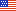An official website of the United States government

# Introduction to Data Visualization in R: ggplot

Introduction to Data Visualization in R: ggplot in R is the fourth class in the NIH Library Introduction to R Series. A basic understanding of R and R Data Types is expected. This class provides a basic overview of producing scatter plots, boxplots, and time series plots using ggplot. R is a programming language and open source environment for statistical computing and graphics. The R class series is a comprehensive collection of training sessions offered by the NIH Library Data Services Program that is designed to teach non-programmers how to write modular code and to introduce best practices for using R for data analysis and data visualization. Each class uses both evidence-based best practices for programming and practical hands-on lessons.

By the end of this class students should be able to: understand the basics of plotting in ggplot; demonstrate how to add a layer to a plot using ggplot; define ggplot aesthetics; add a geometric function to a plot; produce boxplots, and time series plots using ggplot; set universal plot settings; describe what faceting is and apply faceting in ggplot; modify the aesthetics of an existing ggplot plot (including axis labels and color); build complex and customized plots from data in a data frame; exporting a plot using ggplot.

Students are encouraged to install R and RStudio and download the class data before the class so that they can follow along with the instructor. Attendees will need to download the class data before the class.

### Upcoming Sessions

Title Date Times Register

No Sessions Scheduled